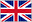# Lecture 05: Lexical Analysis – Deterministic Finite Automaton

0    12 flashcards

QuestionAnswerwhat are the responsibilities of the lexical analyser
 tokenizing source  removing comments  saving text of identifiers, numbers, strings  saving source locations (file, line, column) for error messages
whata re the 3 ways to build a lexical analyser
(1) Write a formal description (e.g., REs) of the token patterns, a Design a state transition diagram (DFA) that describes the token patterns and (2) write a program to implement the diagram, or (3) write a table-driven implementation of DFA.
Whata re state transition diagrams?
directed graphs, are representation of mathematical “machines” called finite state automata (FSA) or just finite automata (FA).
Finite automata could be _____(DFA) or ______(NFA).
Finite automata could be deterministic (DFA) or nondeterministic (NFA).
when is FA determanistic?
A FA is deterministic if it performs the same operation (state transition) in a given situation (its current state and input).
when is fa nondeterministic
A FA is nondeterministic if it can perform any of a set of state transitions in a given situation.
what are the properties of a finite state automaton (FSA), or simply finite automaton
 read in' str' from L-R, 1 sym' at a time in 1 of a finite No. ofState4 each sym' it moves to a new state determined by its current state&the sym' read str' is acptd or rejcted depending on the state of the machine after read the final sym'
what is the formal deffinition of finite automation (FA) M
finite set Q of states, finite alphabet Σ of input symbols, distinguished start state q1 ∈ Q, set of final states F ⊆ Q, and transition function δ: Q × Σ → Q that chooses a new state for M based on the current state, Q, and the current input symbol, Σ
what is DFA for Lexical Analysis
As far as lexical analysis is concerned, a DFA is a string processing machine
what does DFA do for Lexical Analysis
fuck you
what is The set of all strings accepted by a DFA known as
language recognised
how are DFA digrams simplified?
Similar to the case of REs, we name a set of symbols letter = {a, b, c,..., z, A, B, C,..., Z} digit = {0,...,9} and replace their arcs by a single arc labelled letter Or digit (or simply d as in our example)